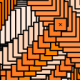# Circles, Spirals and Sunflowers, Part 2

28 Oct

At the end of Part 1, I asked if you could figure out how to draw a spiral, given the code to plot the points in a circle. Have you figured it out? The basic idea is that you change the value of the larger radius (what I was calling lg_rad) as you draw each point. Take a look at the pertinent lines of code in example 6. In this example, as each dot is drawn, at an ever increasing radius, I also increment the angle by just 2 degrees.

We can tighten the spiral by changing the amount we increment the angle during each step, as in example 7. Hit the play button to see the effect. In this example, we start at a 2 degree increment, and then increase the angle by 1 degree per second (since there are 100 dots, the outer dot travels at 100 x 1 degrees or 100 degrees per second).

Watch the above animation for a couple minutes: interesting things happen!. You will notice after about 25 seconds, when the spiral has tightened up, the dots form a spoked or starfish-like pattern. Then the arms fold in, and the dots form a rose-like configuration. Then the arms straighten out again, and you see another starfish. This keeps happening. When the starfish has four arms, the angle increment is 90 degrees, or some multiple of 90 degrees like 270 degrees. The smallest angle that will give you 4 arms is 360/4 or 90 degrees. Similarly, the smallest angle that will get you 5 arms is 360/5 or 72 degrees. 360/6 gives you six arms, 360/7 gives you seven arms and so on. In other words, to get those starfish patterns, the angle increment has to be a rational fraction of (or “go evenly into”) the full circle (so they can line up to form arms).

Between those starfish, which are produced by rational fractions of the circle, you get the rose-like patterns, in which the dots don’t make straight spokes. You get these rose patterns when the angle increment is an irrational fraction of the full circle. Mathematicans have demonstrated that there are far more irrational numbers than rational ones, and intuitively, you can see that there are more roses than starfish in the animation.

There are a number of irrational angles that look especially nice (try the square root of 2 times π or 4.442). The one that produces the most optimal packing, and corresponds to the familiar sunflower arrangement is an angle of approximately 222.5 degrees (or 137.5 going the other way). This is the golden angle, and it is closely related to φ (phi), the golden ratio.

More precisely:

φ = (sqrt(5) + 1) / 2 – 1
golden angle = 360 φ degrees, or 2 π φ radians

If we use that angle to produce a spiral, we get one of these! Example 8 is sometimes called a fibonacci spiral, because the golden ratio is closely related to fibonacci numbers. I also call these phyllotaxy spirals, because the golden angle appears a lot in plant growth (it optimizes surface area to sunlight). Once you start noticing it, you’ll see it in a lot of plants in addition to sunflowers, such as pine cones and agaves.In the above example, you may have noticed that the dots are kind of tight in the center, and then get progressively further apart as they go out. This is because the amount we are increasing the radius is constant, or linear. It would be cool if we could figure out how to get them to pack tightly, like the seeds of a sunflower. It turns out, we already have the mathematical tools to accomplish this!

Consider a large circle which is made up of a bunch of tightly packed little circles – circles so tightly packed that no space remains. If all those little circles are the same size, and the area of the large circle is A, then the area of the little circles, B = A/N, where N is the number of little circles.

As we grow a phyllotaxy spiral, at each step M from 1 to N, we make something very like a circle made up of M tiny circles. The area of that circle is B*M. Since we know that area = 2 π R, we can deduce the desired radius from the area. R = sqrt( area / π )

We can use this technique to figure out (given the number of circles we wish to draw, and the size of the outer circle) both the size of the small circles, and how far out to draw each one. The math for working out the expanding radii isn’t perfect, because there is a little space left over after the cicles are packed together. To compensate for that, we use a fudge factor which keeps the circles from overlapping by drawing them slightly smaller. I find drawing 87% of the perfect size works fine. This produces this figure.

To get even closer to a sunflower, we can make the circles grow larger as they grow outward. One way to easily accomplish this is by making the outer circles grow exactly the same amount as the inner circles shrink, so that cumulatively, the area of the circles remains the same. Here is an example.

Finally, we can use the processing variable frameCount to produce some cool animation effects with these patterns. Click and scroll to the bottom of example 11 for more info about how I did the color cycling. Have fun!

### 2 Responses to “Circles, Spirals and Sunflowers, Part 2”

1.Justin November 11, 2011 at 3:41 pm #

Good tutorial! I made a Spiral Layout class a while ago so that I can get these calculations easily in any sketch that I want.

•jbum November 11, 2011 at 4:26 pm #

Congratulations, you are the first commenter on this blog. :)